# Estimation of Forward Curves

## A First Expedition into the World of Electricity Prices

Preparation

``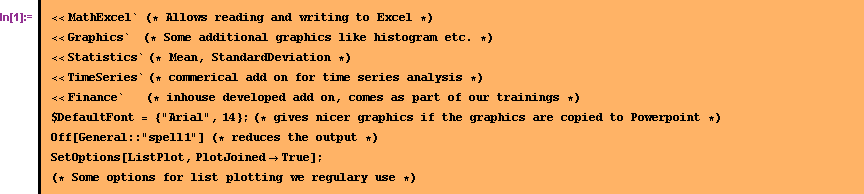``
`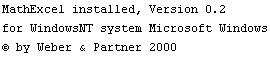`

The prices for electricity we want to analyze are provided from the Leipzig Power Exchange (LPX) as an Excel Sheet. The sheet can be downloaded from http://www.lpx.de/info_center/downloads/dl_general/LPX_Results.xls . To get started the file is opened with Excel and Mathematica is linked to the Excel sheet.

````
`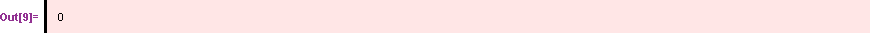`

### A first look at the data

The first column within the Excel sheet contains the dates. These dates will come over the link as OLEDate(s). To get the typical Mathematica representation of dates these OLEDates are transformed to lists. (If we find time we should automate the selection of the range. At the moment the range is hard coded.)
In the Excel sheet the first data row is the most recent one. For time series analysis it is more convenient to have the most recent observation as the last element. The reordering of the data can be achieved with the Mathematica command Reverse. Since the first day in the historical data set starts with prices of zero for some unknown reasons, we just throw away this observation with the command Rest.

````
``

In the second column of the Excel sheet are the prices for the base load.

``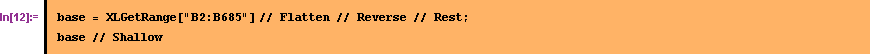``
``

To get a first impression of the data we plot the data, doing some adjustments to the regular plot options and providing non-standard ticks.

````Ther are large jumps observable in December 2001. At the moment we are not interested in these jumps therefore we use another scale in the plotting.

``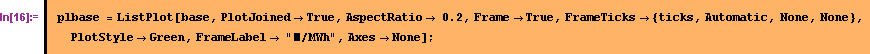``There is no up- or downward trend recognizable in the data. Remarkable are the repeating patterns in the data. Observable are also jumps with different magnitude, whereby the level comes back very fast after a jump.

The third column within the Excel sheet has the prices for the peak load.

````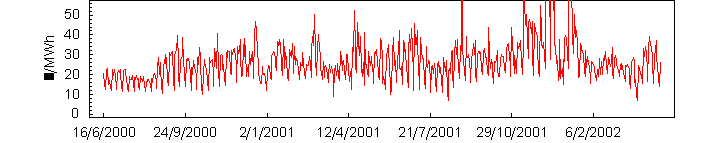The plot for the peak load looks at first sight very similar to the plot of the base load. The similarity will be analyzed later.

In the Excel sheets are also the prices for every hour.

````
`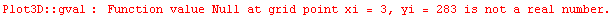`
``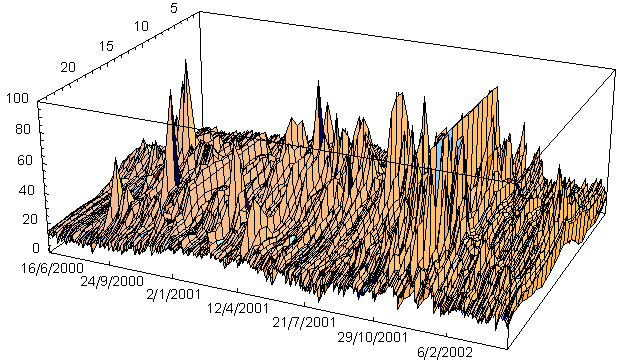The warning from Mathematica tells us that some prices are missing. We will ignore this message for the moment. It looks like there are a lot of  jumps in the hourly prices. Also it looks like there is a typical pattern over a day. To further explore this pattern over a day the mean for each hour is calculated and plotted.

````
`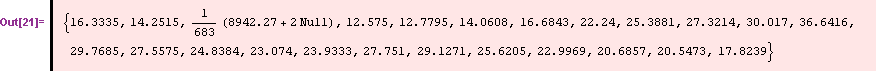`#### Comparing base and peak load prices

To further explore the seemingly similarity of the peak and base load prices both time series are plotted in one graph together with the differnces between them. As expected the prices of the peak load are higher then the base load in most cases.

``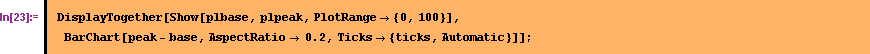``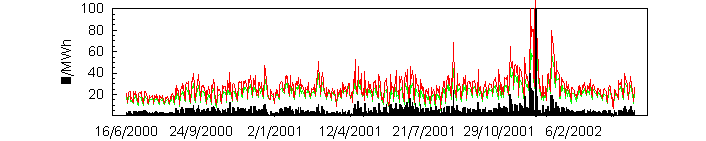But there are some exceptions to this rule

``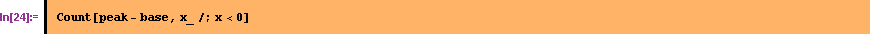``
`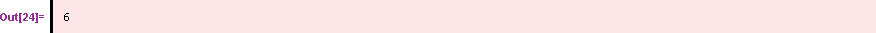`

The correlation which can be sensed from a look at the data is confirmd through a regressionen analysis. For the regression analysis we look at the one day changes of the prices.

````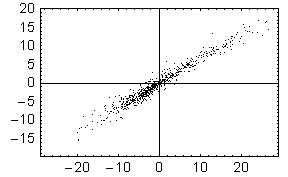``

Interesting is always the look at the residuen of the regression.

````A mean to explore through visualization the possible deviation of the observed distribution to the normal distribution is the QQ-plot. The QQ plot is not available in Mathematica but it can be constructed with a few lines of Mathematica code.

``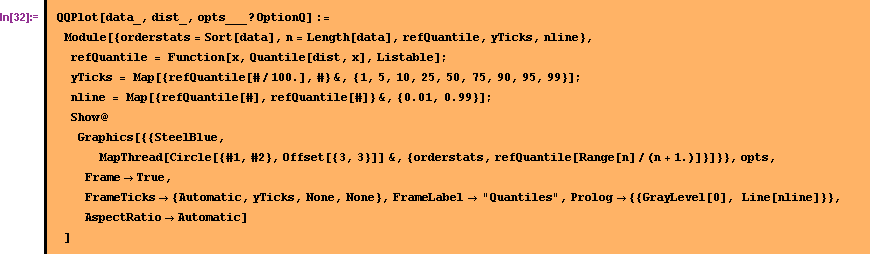``

Applying the QQ plot to the residuals shows us that the distribution of the residual is not a normal distribution. If it would be, the blue circles would be closer to the straight black line.

````#### Looking for auto correlations

We are also interested in possible auto-correlations in the data. To visualize them we can build an autocorrelation plot, which is constructed around the function CorrelationFunction which comes with the package TimeSeries.

````

The doted gray lines are the boundary at which autocorrelations are significant at a 95% level.

##### Day-of-week effects

The newly constructed plot can be used for analyzing the data. As a first analysis the one day differences in the data are explored. The function Difference comes from the TimeSeries package.

``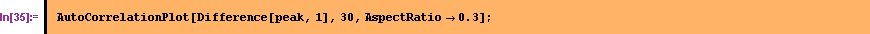``There seems to be significant autocorrelation in respect to the previous day, the day before the previous day and then on the seventh,day. This indicates a day-of-the-week effect in the data.

##### Seasonal effects

If we only look on weekly sequences of the data a seasonal pattern can be observed.

````
``
``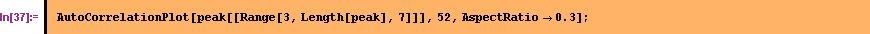``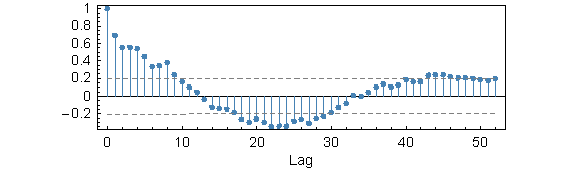When looking at all the sundays in the data there is a significant negative autocorrelation around the 26th week, indicating a half year effect. It is interessting that these autocorrelation are not so clearly visible for other days of the week (yet?)

``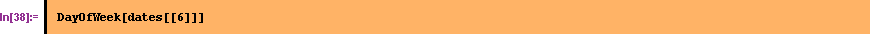``
`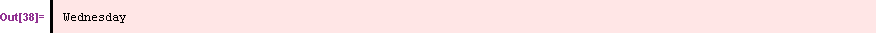`
``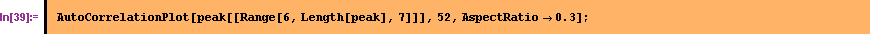``### A first try to construct a forward curve

We have the feeling that parts of the price changes are not random, i.e we think we are able to predict in part the future evolvement of the prices. To achieve this we try to identify the non-random parts of the data and build a function for them. We call this function a forward curve. Our assumption is that the future prices will randomly realize around this forward curve.

Doing an autocorrelation analysis and looking at the data seemed to reveal an day-of-the-week effect. To further explore this day-of-the-week effect, the data is rearranged in weekly chunks, then the mean for each week is calculated and the difference of each day of the week to the mean of the week is calculated.

``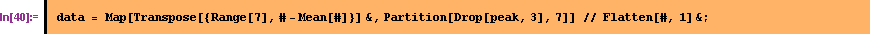``

This results in the following picture. The x-axes describe the week days starting with Monday and ending with Sunday. As expected are the prices on Sunday below the average of the week.

``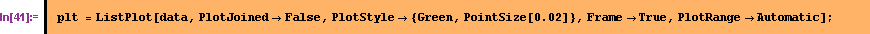``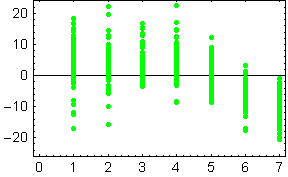To fit a curve into this data we use our usual first template for non-linear estimation.

``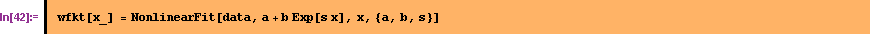``
``

In this case the function seem to able to follow the data.

````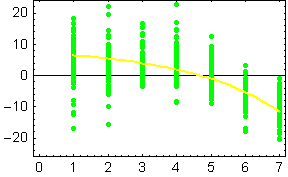We then use the function to "correct" the observed prices. The RotateLeft is necessary because the data does not start with a Monday.

``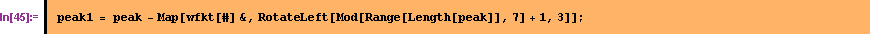``

The autocorrelation plot for the "corrected" data reveals that the weekly autocorrelation has disappeared.

``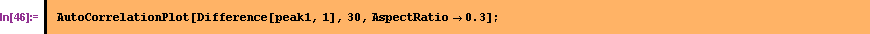``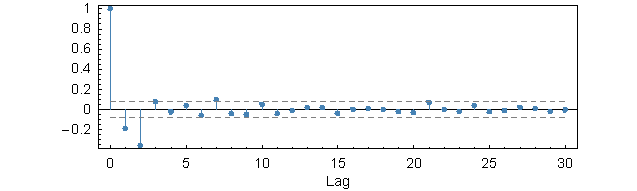#### Controlling for holidays

From the observation, that Sundays have special prices one might also conclude special prices on holidays. To explore this idea first we use the HolidayQ function which comes with our package Finance, which was loaded in the beginning. We do not have a holiday calendar for Leipzig available so we take Frankfurt instead.

``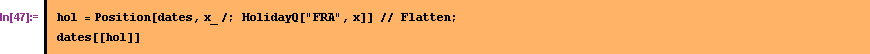``
``

Marking the holidays in the time series with a green point convinces us that our conclusion about holidays is not false:

````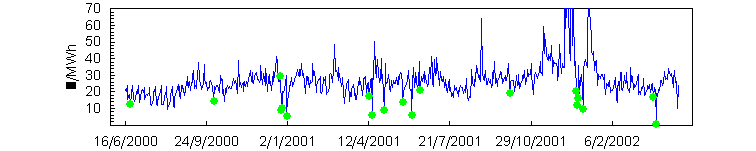We calculate the relative mean price decrease due to a holiday.

````
`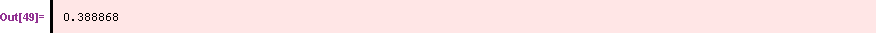`

and the relative price increase after a holiday.

``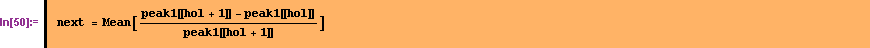``
`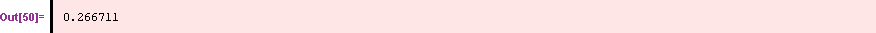`

The simplest approach controlling for holidays is to get rid of them. Therefore we throw them away

``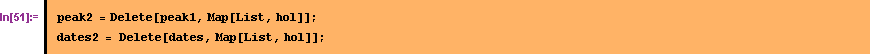``

#### Estimating seasonal effects

In the autocorrelation analysis were seasonal effects visible at least when looking on the Sunday's prices. Other studies of electricity prices from other countries also regulary find seasonal pattern. To estimate seasonal patterns the dates are expressed as fractional years.

``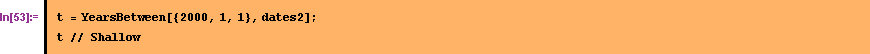``
`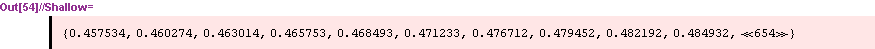`

and the prices are calculated relative to the mean.

````
`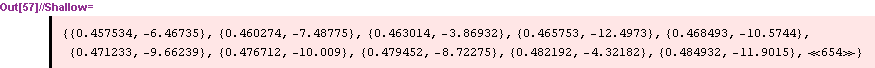`

For estimating a functionf for the seasonal effects a non-linear curve is fitted on this data. The template for the function was taken from Pilipovic (1997).

``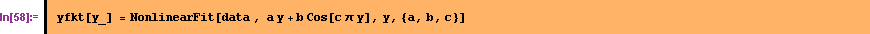``
`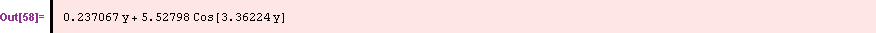`

To compare visually the estimated curve with the data the following graph is constructed

````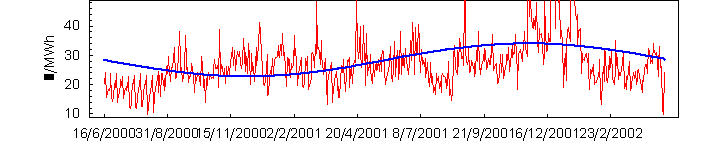Fur further analysis (not shown in this notebook) the estimated function for seasonality is used to further adjust the observed prices.

``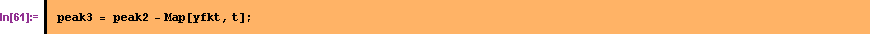``

#### Constructing the forward curve

Using the results of the previous analysis a forward curve can be constructed for the valuation date. It is assumed that the valuation date is the next to the last observed date. The forward curve should start at the last observed price, assuming that the last price together with the above estimated corrections is the best estimation for the future prices.

````
``
`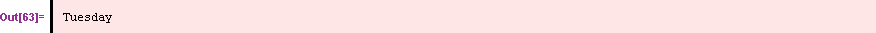`
`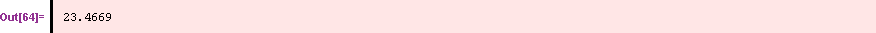`

The dates for the coming 365 days are constructed and the day of the week and the year fraction for each day are calculated.

````

Together with the above estimated curves a forward curve can be constructed.

``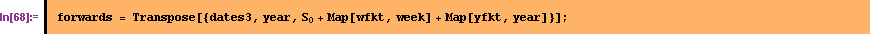``

To visualize the estimated forward curve it is added to the plot of historical prices.

``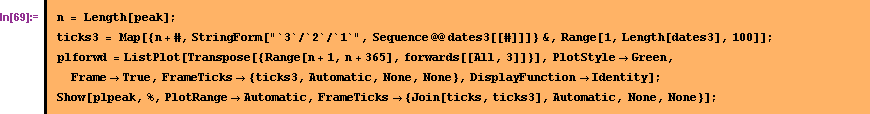``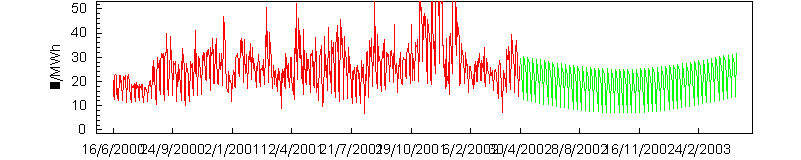### Summary

This example for estimating forward curves are a first expedition into the world of electricity prices. The analysis was done in preparation for a project. Doing this exercise helped us to understand more sophisticated approaches for estimating forward curves. Several parts are missing in this notebook like the estimation of volatilities, adjusting the forward curve to trade prices, controlling for jumps etc. Eventhough we left out a lot of important topics  we hope that this example is usefull as a starting point for others.

### References

Cleveland, William S., (1993), Visualizing Data, New Jersey 1993.

Clewlow, Les/Strickland, Chris, (2000), Energy Derivatives: Pricing and Risk Management, Lacima Publications.

Pilipovic, Dragana, (1997), Energy Risk: Valuing and Managing Energy Derivatives, McGraw-Hill.

Converted by Mathematica      April 29, 2002